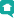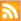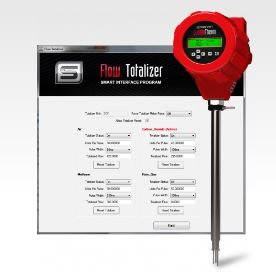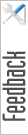Blog HomeRSS Feed

## Official Blog of Sierra--Let's Talk Flow!

##### Topics

Subscribe to our mailing list to get news about new products, application stories, & more!

# Understanding the iSeries Totalizer for Gas Mass Flow Rate

Jan 23, 2018Do you have questions about the iSeries flow meter Totalizer App that comes standard with every QuadraTherm 640i/780i Thermal Mass Flow Meter or want to learn more about gas mass flow rate? We get many support questions regarding the app, generally covering the same few points, so let’s spend a few minutes and discuss how to use and understand the power of the iSeries flow meter Totalizer.

## What is a Flow Meter Totalizer

Simply put, a totalizer is a running total of how much fluid (gas, liquid, steam) has passed by the sensor within a given time. For example, “in the last 60 seconds, there have been 30 gallons of water flowing by the sensor.”

Along with the totalizing of the flow, there are additional features available for the iSeries Totalizer:

1. Activate a relay pulse whenever a known quantity of flow has passed over the sensor. An example of this would be, “pulse the relay for 50ms whenever we flow 2 gallons.”

2. Activate a relay if the totalizer value is less than (Low Alarm), more than (High Alarm), or outside a low and high value (Window Alarm). An example of this would be “Activate the alarm if we exceed 30 gallons of totalization.” This would be considered a “high alarm.”

3. Do not totalize if the flow exceeds fullscale, utilizing the Totalizer Clipping option.

## Understanding Units Per Pulse

Let’s say that our fullscale is 600 gallons per minute. When we’re speaking about fullscale, this means the maximum flow we expect, not the current flow. Say we want to pulse the relay for every 20 gallons that have passed by the sensor. There is a setting in the totalizer screen called the “Units Per Pulse” (UPP).

So, back to our example. We have a fullscale of 600 gal/m (gallons per minute), and we want to totalize and pulse the relay every 20 gallons. Setting a value of “20” in the UPP field tells the meter that we want to totalize and pulse every UPP amount, or in this case, every 20 gallons.

That’s it!

well, kind of.

It’s the “kind of” part that causes us headaches!

## What Causes Totalizer Headaches and How to Solve Them

In the current release of the iSeries Raptor 2 platform (version 1.x), we have a historical rule that states that we cannot pulse the relay more than 1Hz, meaning that we cannot pulse the relay more than once per second. This is a carryover from the older products and was honored with the v1.x of the Raptor platform to be backward compatible.

We need to ensure that we do not pulse more than once per second. Said another way, the UPP value cannot be such that it would “break” the 1Hz rule. So how do we account for this? Simply put, all we need to do is determine what would be the lowest possible UPP value that would not break the 1Hz rule. To do that, we just have to make sure that the value we use is equal or larger than that value.

For our fullscale of 600 gal/m, we would first determine what the UPP value would be for a valid 1Hz pulse. This is done by simply figuring out what our fullscale flow would be per second. Since our fullscale is 600 gal/per minute, we just take that value of 600 and divide by 60 to make into seconds. So 600/60 = 10. So that means at fullscale flow, we will be flowing 10 gal/second. So, for our UPP value, we must use a value equal to or greater than 10.

If we were flowing 600 gal/per hour, then we would divide 600 by 3600 (60 seconds * 60 minutes) = 0.1666. We must ensure that the UPP value is equal to or larger than that.

Rule: A valid UPP *must* be greater than or equal to the fullscale value converted to seconds

So, what would happen if you entered a UPP value that breaks the 1Hz rule? For example, what if instead of entering a 10 for the UPP (10 = 1Hz), you entered a 5 for the UPP (5 = 2Hz, 2 times per second)?

Well, the totalizer updating would not be affected. It would still correctly totalize the flow. The issue would manifest itself in the physical pulsing of the relay. It’s the relay pulsing that honors the 1Hz rule. To do that, it will continue to pulse at 1Hz, but will buffer/remember that it still needs to keep pulsing. For our working example of UPP=10, we would pulse the relay 60 times per minute (if flowing at fullscale). Using the wrong value of UPP=5, we would need to pulse the relay 120 times per minute, but since we are honoring the 1Hz rule, it will actually take 120 seconds. What that means is if we were to go to zero flow after 1 minute, we have only pulsed 60 pulses of the 120 we need to do; we would continue to pulse the totalizer relay, even if we have no flow!

If you ever “see” the totalizer pulsing after the flow has gone, you have entered the wrong UPP value, or you are flowing beyond the fullscale value. If you are flowing beyond the fullscale, you could activate the “Force Totalizer Clipping” functionality to stop it.

What happens if you don’t want to totalize if the flow exceeds the fullscale value? Easy, we have a checkbox in the totalizer screen that tells the meter not to totalize the flow if it exceeds the fullscale.

Once the flow returns to fullscale or lower, the totalizer continues to work “as normal.”

Understanding Pulse Width

The Pulse Width is the time duration that the relay is active for. Entering a value of “50ms” (milliseconds) means that we will pulse the relay for 50ms. The user can select the duration of the pulse. You cannot exceed 1000ms (1 second) per pulse.

## More examples

Let’s look at a few more examples to illustrate how we determined the UPP value.

Example 1:

Fullscale is 5 MMSCFD

We want the totalizer to pulse every 1000 SCF

Let’s calculate the minimum UPP we can use, just to make sure we don’t violate the 1Hz rule. Since our fullscale is 5 MMSCF D (per day), we divide it out until we get seconds. UPP = 5 / 86400 (60 sec * 60 min * 24 hours = 86400 seconds) = 0.00005787 MMSCF S (per second). We now know that whatever value we use for the UPP, it must be equal or greater than this value to honor the 1Hz rule.

Now we want to pulse the totalizer every 1000 SCF. Since we are in MM units for the fullscale, we must convert the 1000 to MM to make both numbers equal regarding units. 1000 SCF = 0.001 MMSCF. So, setting the UPP=0.001 tells the meter to pulse the totalizer every 0.001 MMSCF units, or every 1000 SCF. Since the 0.001 value is greater than the minimum UPP of 0.00005787, we have not violated the 1Hz rule.

Fullscale is 5 MMSCFD

We want the Totalizer to pulse every 1000 SCF

Set the UPP=0.001

It should be noted that the UPP value must be in the same units as the fullscale. Since we are in MM units, the value in the UPP must also be in MM units; hence why the 1000 = 0.001 (MM).

Example 2

Fullscale is 22,000 gal/h

We want the Totalizer to pulse every 5 gal

Let’s calculate the minimum UPP. 22,000 gal/h = 22,000/3600 (60 sec * 60 min) = 6.1111. So our minimum UPP cannot be lower than 6.1111 gal for the 1Hz rule.

We want to pulse the totalizer relay every 5 gal. Setting the UPP = 5 would not work as the minimum UPP is 6.1111! Tricked you! This is a bad example.

So as you can see, we cannot do a relay pulse every 5 gallons as it violates the 1Hz rule.

Example 3:

Fullscale is 22,000 gal/h

We want the totalizer to pulse every 15 gal

Since the 22,000 and the 15 are in the same units (meaning they’re not MM nor M), we can leave the numbers alone. We set the UPP=15 to indicate we want a pulse every 15 gallons that have flowed by.

Fullscale is 22,000 gal/h

We want the totalizer to pulse every 15 gal

Set the UPP=15

## Be a Flow Totalizer Expert

That’s it. Keep the formula in mind, and you should be a full-fledged, flow totalizer expert.Written By:
Kam Bansal, Dir. of Engineering
Sierra InstrumentsSubmit a Bug Report

Name

Email

Reproducibility (Did you try to reproduce the bug?)

Classification

Summarize the problem but be specific enough that it can be recreated.

Note: Page, browser, and OS are automatically saved